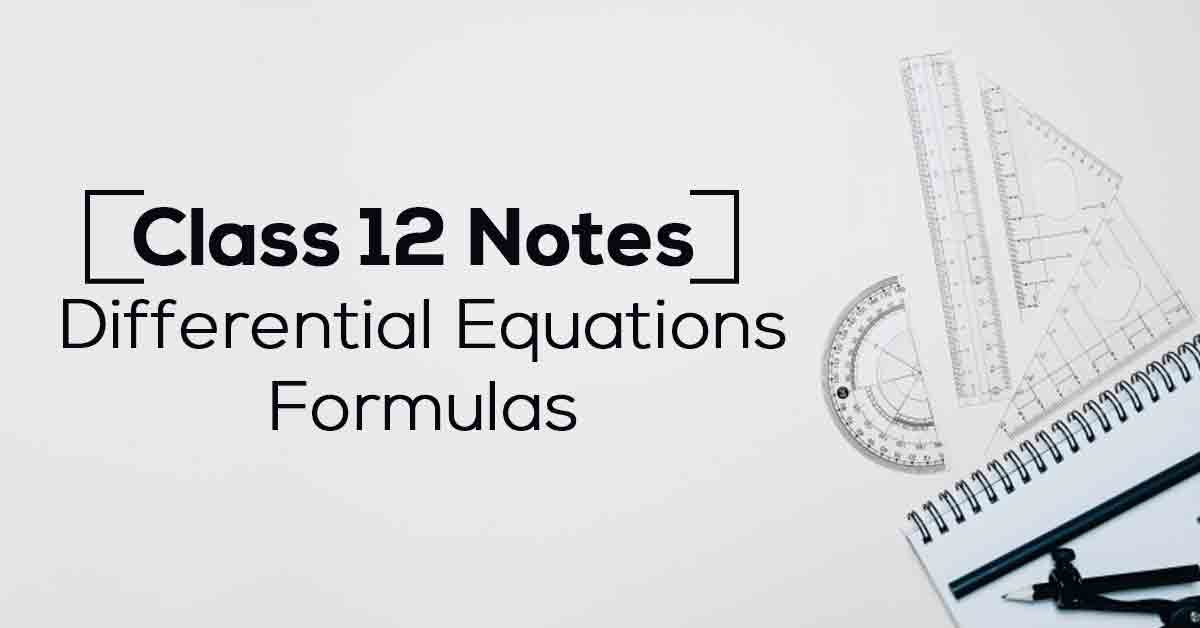Class 12th Math Differential Equations Formulas CBSE 2023

# Differential Equations Class 12 Formulas PDF with Notes## Class 12 Maths Chapter 9 Differential Equations Formulas – PDF Download

A differential equation is a mathematical equation that relates some function with its derivatives. In applications, the functions usually represent physical quantities, the derivatives represent their rates of change, and the equation defines a relationship between the two. Know More about these in Differential Equations Class 12 Formulas List.

The topics and sub-topics covered in Differential Equations Class 12 Formulas are:

9.1 Introduction

9.2 Basic Concepts

9.2.1. Order of a differential equation

9.2.2 Degree of a differential equation

9.3. General and Particular Solutions of a Differential Equation

9.4 Formation of a Differential Equation whose General Solution is given

9.4.1 Procedure to form a differential equation that will represent a given family of curves

9.5. Methods of Solving First Order, First Degree Differential Equations

9.5.1 Differential equations with variables separable

9.5.2 Homogeneous differential equations

9.5.3 Linear differential equations.